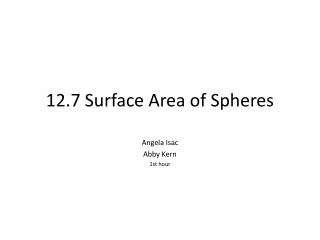DownloadDownload Presentation12.7 Surface Area of Spheres

# 12.7 Surface Area of Spheres

Télécharger la présentation## 12.7 Surface Area of Spheres

- - - - - - - - - - - - - - - - - - - - - - - - - - - E N D - - - - - - - - - - - - - - - - - - - - - - - - - - -
##### Presentation Transcript

1. 12.7 Surface Area of Spheres Angela Isac Abby Kern 1st hour

2. Objectives • Recognize and define basic properties of spheres. • Find surface areas of spheres.

3. What is a Sphere? • A sphere is the locus of all points that are a given distance from a given point called the center. • To help visualize a sphere, consider infinitely congruent circles in space that all have the same point for their center.

4. Properties of Spheres • A radius of a sphere is a segment with endpoints that are the center of the sphere and a point on the sphere. In the figure, DA, DB, and DC are radii. • A chord of a sphere is a segment with endpoints that are points on the sphere. In the figure, GF and AB are chords. • A diameter is a chord that contains the center of the sphere. In the figure, AB is the diameter. • A tangent to a sphere is a line that intersects the sphere in exactly one point. In the figure, JH is a tangent to the sphere at point E.

5. Great Circles • When a plane intersects a sphere so that it contains the center of the sphere, the intersection is called a great circle. • A great circle has the same center as the sphere, and its radii are also radii of the sphere.

6. Hemispheres • Each great circle separates a sphere into two congruent halves, each called a hemisphere. • A hemisphere has a circular base.

7. Example #1 In the figure, O is the center of the sphere, and plane R intersects the sphere in circle A. If AO = 3cm and OB=10cm, find AB.

8. Example #1 Cont. Use the Pythagorean Theorem to solve for AB. OB2 = AB2 + AO2Pythagorean Theorem 102 = AB2 + 32OB = 10, AO = 3 100 = AB2 + 9 Simplify. 91 = AB2 Subtract 9 from each side. 9.5 = AB Use a calculator. Answer: AB is approximately 9.5cm.

9. Surface Area of Spheres If a sphere has a surface area of T square units and a radius of r units, then T= 4 r2 This is simply saying that the surface area (T) of the sphere is 4 times the area of the great circle ( r2).

10. Example #2 Find the surface area of the sphere given the area of the great circle.

11. Example #2 Cont. Use the formula for surface area to solve. T = 4 r2Surface are of the sphere. T = 4(201.1) r2 = 201.1 T = 804.4 Multiply. Answer: 804.4 in2

12. Area of a Hemisphere Since a hemisphere is half a sphere, to find its surface area, find half of the surface area of the sphere and add the area of the great circle. T = ½(4 r2) + r2

13. Example #3 Find the area of the hemisphere.

14. Example #3 Cont. Use the formula for the surface area of a hemisphere to solve. T = ½(4 r2) + r2 Surface are of a hemisphere T = ½[4 (4.2)2] + (4.2)2 Substitution T = 166.3 Use a calculator. Answer: 166.3cm2

15. Assignment Pre-AP Geometry: Page 674 #10 - 29